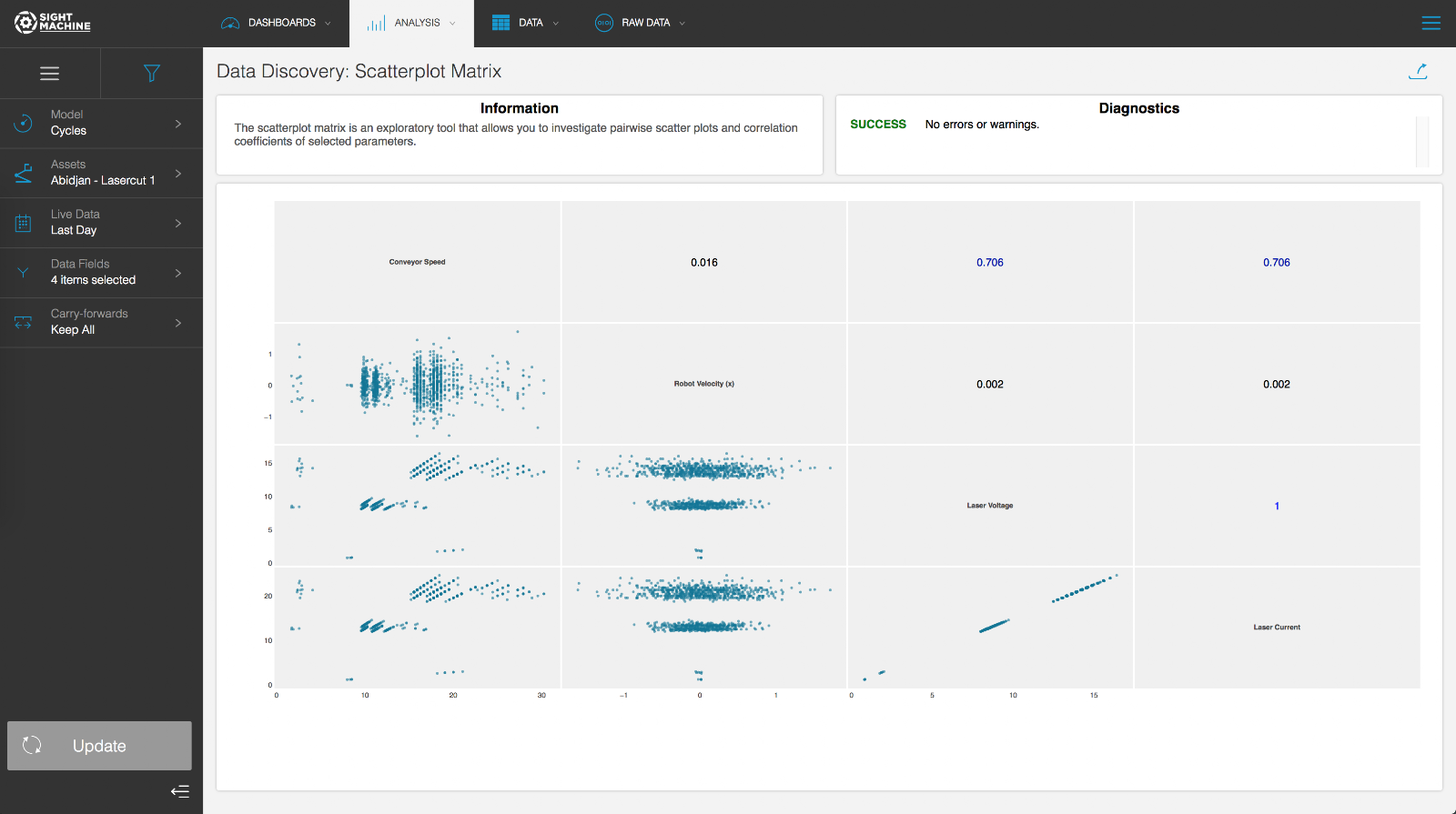Scatterplot MatrixUse Cases

The Scatterplot Matrix plots the pairwise scatterplot for all combinations of the selected parameters. It is designed for comparing multiple parameters at the same time, with a greater level of detail than the Correlation Heatmap and with more breadth than the Curve Fit Analysis. This tool is most helpful early in the exploratory process to identify patterns that you want to investigate in more detail. This is a good tool to lead to Curve Fit Analysis.

Specifications

The Scatterplot Matrix is a multivariate analysis that you can perform on continuous fields on the Cycle and Part models. You can select multiple machines of a given type when the analysis is performed on the Cycle model. Using the Cycle model, the Scatterplot Matrix can investigate parameters from the same machine type with high granularity. Using the Part model lets you compare fields across different machines, but with lower granularity (the Part model is limited by the serial number or other identifier used to construct the data model).

Analysis

The Scatterplot Matrix allows you to investigate the pairwise relationships among up to 10 parameters at the same time. The downward diagonal in the matrix labels the parameters. For example, the lower-left corner plot shown is Conveyor Speed (X axis) vs. Laser Current (Y axis). The lower-left side of the matrix plots the scatterplot for each parameter combination. The upper-right side of the matrix gives the Pearson-R correlation coefficient for each pair.